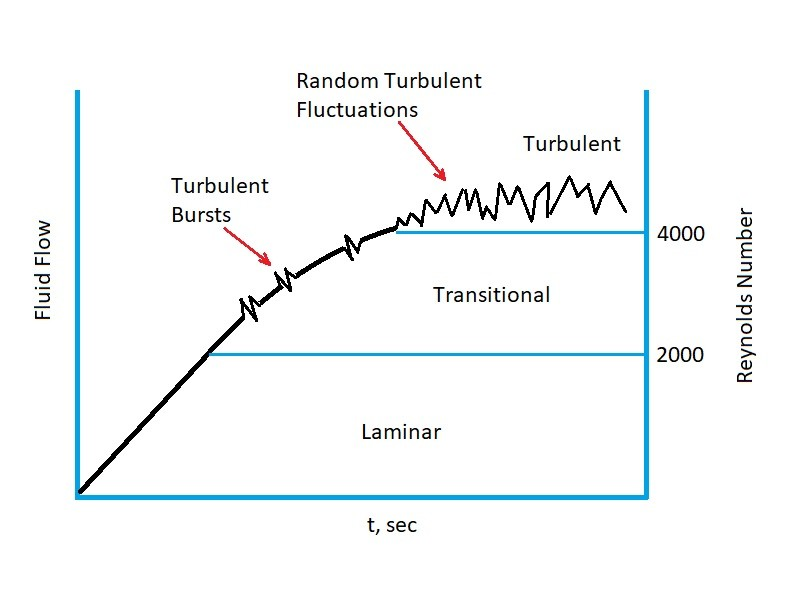# Transitional Flow

Normally a flow is either classified as laminar or turbulent.  However, there is a transitional phase where flow can experience both laminar as well as turbulent characteristics.  When this occurs the fluid is transitioning between laminar flow and turbulent flow.  In other words it is in a state of transitional flow.

Reynolds number is a dimensionless number that is used to determine whether a fluid’s flow is laminar or turbulent.  For pipe flow, a flow is considered laminar if Reynolds number is less than 2100.  On the other hand if Reynolds number is above 4000 than the flow is considered turbulent.  Anything in between these two number is in transitional flow. However, these Reynolds number values are only true for pipe flow.  If the geometry for the fluid flow is different, the transition from laminar to turbulent will occur at different Reynolds number values.  For example, for a flat plate the transition from laminar flow to turbulent is around 500,000.

(Eq 1) $Re=\frac{ρvd}{μ}$

$Re$ = Reynolds Number

$ρ$ = fluid density

$d$ = pipe diameter

$μ$ = dynamic viscosity

### Transitional Flow and Reynolds Number

To understand how Reynolds number causes a transition between laminar a turbulent flow, let’s take a look at the following example.  First, at time zero the flow in a pipe will be at rest.  In this state the Reynolds number will also be at zero.  Next, the flow will steadily increase in such a way that unsteady effects will be negligible.  As time passes the flow will increase to a Reynolds number of 2100 and the flow will be laminar.  However, once Reynolds number become greater than 2100, the flow will experience random turbulent bursts while also still behaving like a laminar flow.  At this point the flow has reached transitional flow.  Finally, Reynolds number will increase to 4000 and the flow will become fully turbulent.  The reason that this occurs is because of the viscous effects of the fluid as it flows past the pipe’s wall.|